﻿ SQL Where Clause - TutorialsTrend
This Angular tutorial helps you get started with Angular quickly and effectively through many practical examples.
`

# SQL-Where Clause

In this tutorial we will learn about where statement and how to use where statement in SQL Server.

#### SQL Server WHERE Statement

The SELECT statement in SQL returns either all the columns (if we use *) or specific columns. There are many situations where we want to retrieve records based on certain conditions. This can be achieved using the WHERE Clause.

You can use the WHERE clause as follows:

``````SELECT
column-name1,
column-name2,
FROM
table_name
WHERE search_condition;``````

In the WHERE clause, you specify a search condition to filter rows returned by the FROM clause. The WHERE clause only returns the rows that cause the search condition to evaluate to TRUE.

To understand concept of WHERE we create a table named as students and inserted some data in that table.

Consider the following Student table:

``````SELECT *
FROM students;``````

Table lookes like that: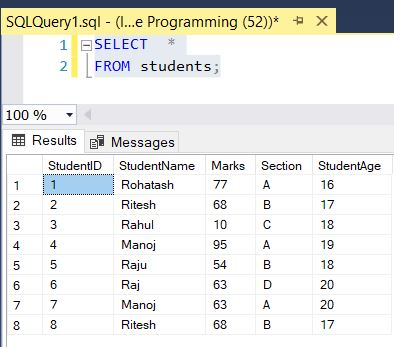#### WHERE with equality(=)

The following statement retrieves all studentname with the section is A:

``````SELECT StudentID, StudentName, Marks, Section, StudentAge
FROM students
WHERE Section = 'A'``````

Query output looks like that: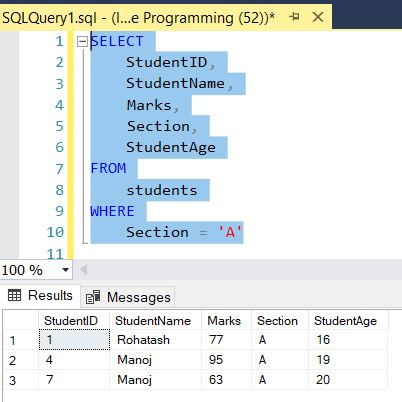#### WHERE with two conditions(AND)

The following example returns products that meet two conditions: Section is A, and the studentname is manoj'. It uses the logical operator AND to combine the two conditions. SQL Server WHERE - match two conditions:

``````SELECT StudentID, StudentName, Marks, Section, StudentAge
FROM students
WHERE Section = 'A' AND StudentName = 'manoj'``````

Query output looks like that: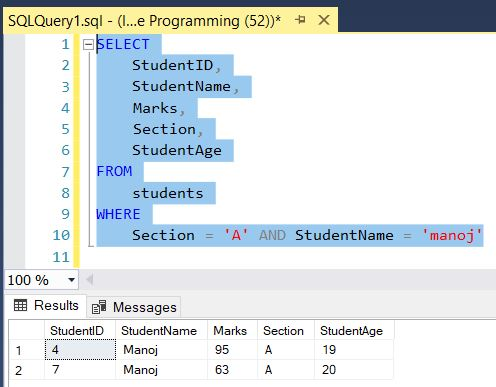#### WHERE with two conditions(OR)

The following example returns products that meet two conditions: Section is A, and the studentname is manoj'. It uses the logical operator OR to meets one of these conditions are included in the result set. SQL Server WHERE - match any of two conditions

``````SELECT StudentID, StudentName, Marks, Section, StudentAge
FROM students
WHERE Section = 'A' OR StudentName = 'manoj'``````

Query output looks like that: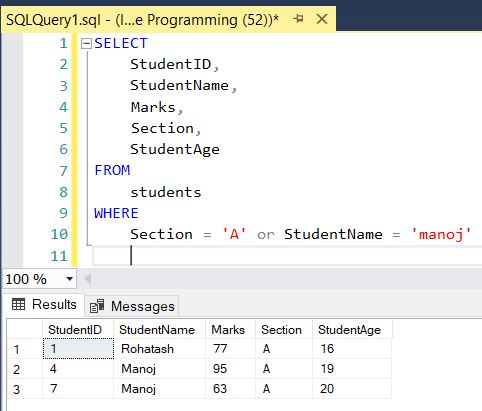#### WHERE with IN Operator

The following example uses the IN operator to find rows that have a value in a list of values. SQL Server WHERE - IN operator:

``````SELECT StudentID, StudentName, Marks, Section, StudentAge
FROM students
WHERE StudentName in('Rohatash', 'Ritesh','Rahul', 'Manoj')``````

Query output looks like that: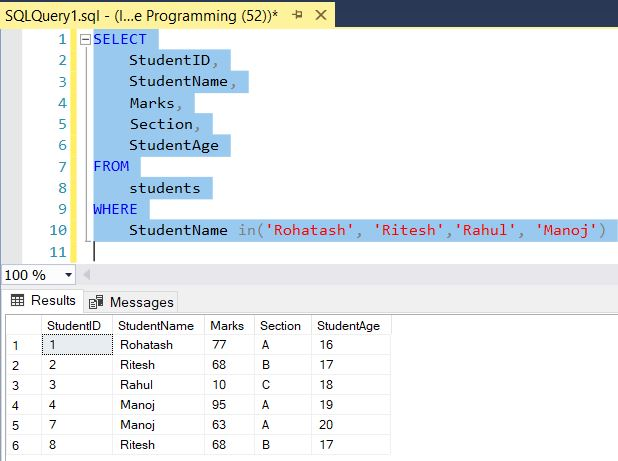#### WHERE with BETWEEN Operator

The following example uses the BETWEEN operator to find rows with the value between two values. The following query which use between operator.

``````SELECT StudentID, StudentName, Marks, Section, StudentAge
FROM students
WHERE marks BETWEEN 63 AND 100``````

Query output looks like that: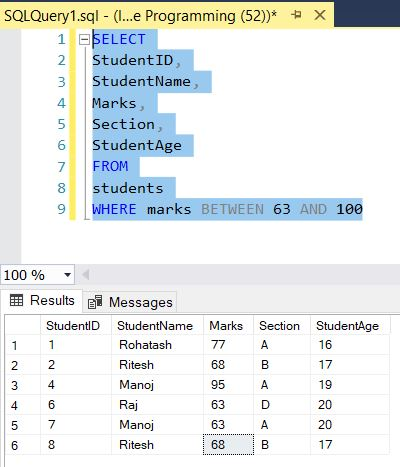#### WHERE with LIKE Operator

The following example uses the LIKE operator to find rows whose values contain a string. The following query which use like operator.

``````SELECT StudentID, StudentName, Marks, Section, StudentAge
FROM students
WHERE StudentName like '%ra%'``````

Query output looks like that: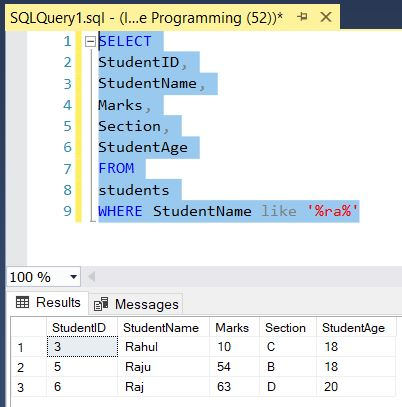Next